## Extraction of knowledge from data using Computational Intelligence methods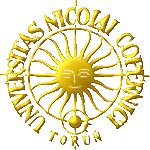### Włodzisław Duch Rafał Adamczak Krzysztof Grąbczewski Karol Grudziński Norbert Jankowski Antoine Naud### WWW: https://www.fizyka.umk.pl/~duch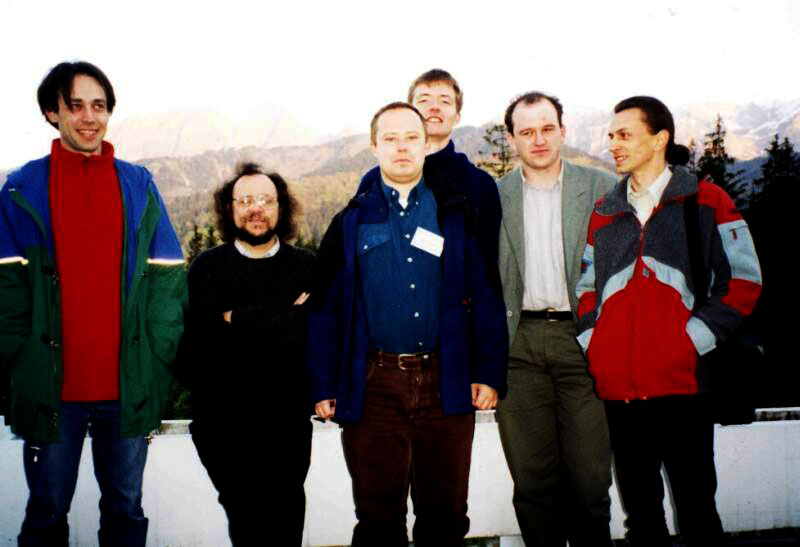## Plan

1. Intro: understanding the data and knowledge discovery

2. Logical explanations: types of rules and their decision borders

3. Prototype-based explanation: SBL, Similarity Based Learner

4. Visualization-based explanation:
5. Some knowledge discovered

6. Example: system for analysis of psychometric questionnaires

7. Open problems

## Understanding the data and knowledge discovery

• how to discover knowledge in the data;
• how to understand what the data mean, create comprehensible model of the data;
• how to evaluate new data.

Neural networks are universal approximators/classifiers (see textbooks for references)
... but are they good tools for real applications?

More methods of classification than datasets to classify.
Computational intelligence (CI) methods developed by experts in:

• statistics, patter recognition, clusterization, discrimination;
• machine learning (ML), inductive rule-based AI methods;
• neural networks;
• logics, fuzzy and rough;
• numerical taxonomy;
• visualization;
• other ...
• Machine Learning camp: black box classifiers (such as NNs) are unacceptable - symbolic rules forever.
• But ... knowledge accessible to humans: symbols, similarity to prototypes, visualization.What type of explanation is satisfactory?
Interesting cognitive psychology (CS) problem.

Exemplar and prototype theories of categorization in CS:

• humans remember examples of each category and refer to such examples
k-NN, k-nearest-neighbors type of reasoning
• humans create prototypes out of many examples;
like Gaussian classifiers, RBF networks

Both are true, logical rules are the highest form of summarization.

Types of explanation:

• logic-based: symbols and rules
• exemplar-based: prototypes and similarity
• visualization-based: maps, diagrams, relations

Best explanation - depending on particular field.

Other implications of knowledge extraction:

• Understanding what Computational Intelligence (CI) system has learned.
• Use of symbolic knowledge in neural networks:
• knowledge-based neurocomputing;
• domain knowledge for initialization may allow to find better solutions;
• pre-structuring or restricting the type of answers.
• Use of distributed representations in symbolic systems for knowledge acquisition, association and generalization.

Use of various forms of knowledge in one system is still rare.
Cf. DISCERN - distributed lexicon, NLP dialog system (Mikkulleinen)

## Logical explanations

Logical rules, if simple enough, are preferred by humans.• Explanations 'why' are in some applications necessary
• Rules may expose limitations of black box approximations.
• Only relevant features are used in rules.
• Rules may sometimes be more accurate than NN and other CI methods.
• Overfitting is easy to control, rules usually have small number of parameters.
• Rules forever!

Are rules indeed the only way to understand the data?

• IF the number of rules is relatively small AND
• IF the accuracy is sufficiently high.
• THEN rules may be   an optimal choice.

Types of logical rules:

1. Crisp logic rules: for continuos x use linguistic variables (predicate functions):
sk(x) ş True [XkŁ x ŁX'k], for example:
 small(x) = True{x|x<1} medium(x) = True{x|x Î [1,2]} large(x) = True{x|x>2}

Linguistic variables are used in crisp (propositional, Boolean) rules:

IF small-height(X) AND has-hat(X) AND has-beard(X) THEN (X is a Brownie)ELSE IF ... ELSE ...

Crisp logic based on rectangular membership functions: True/False values jump from 0 to 1.
Step functions are used for partitioning of the input space.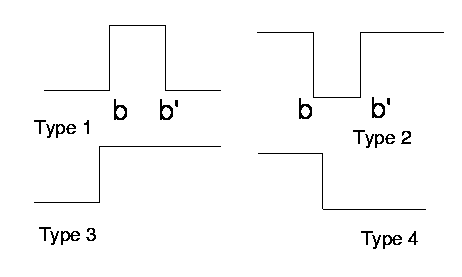Decision regions: hyperrectangular (cuboidal).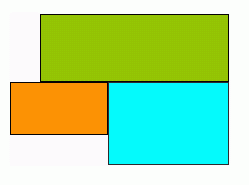For example, some rules for the Iris data are:

 Setosa PL <2 AND PW < 0.6 Virginica PL > 4.9 OR PW > 1.6 Versicolor ELSE

Here are decision regions for these rules.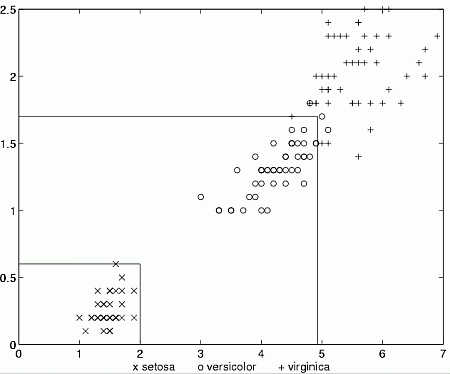Decision trees provide crisp rules applied in a specific order.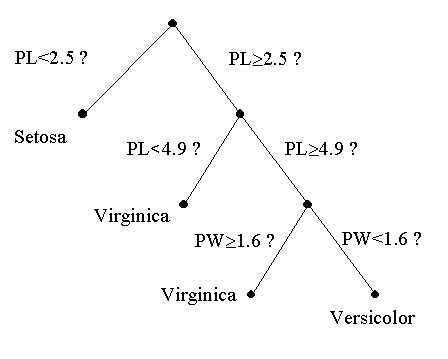Here is a decision tree for Iris data.

Below its decision regions.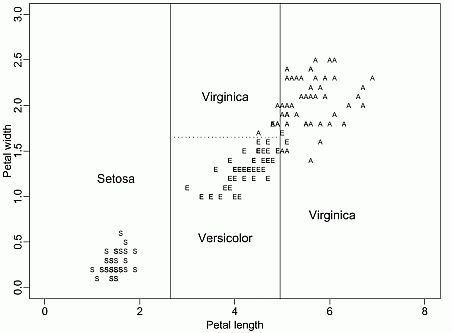If hyperrectangular regions are too simple, rules are not accurate;
Solution: allow linear combinations of some inputs x.
Oblong decision trees, LDA, Linear Discrimination Analysis.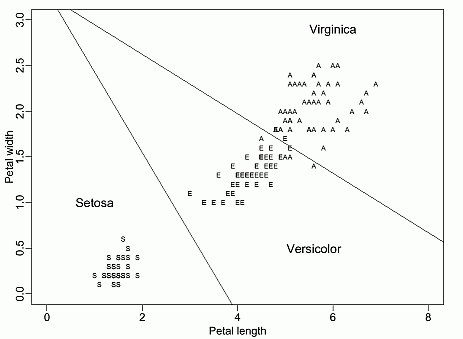For example, a good rule for Iris is:
IF (SL+1.57SW-3.57PL-3.56PW<12.63) THEN Iris-versicolor

The number of problems that one may analyze using crisp logic may be limited.

2. Fuzzy logic rules

Typical approach: define triangular, trapezoidal, Gaussian and other type of membership functions.
Membership function m(x) - degree of truth that x has the property m
Instead of 0, 1 predicate function map into [0,1] interval.

Partition every feature into some membership functions, for example triangular.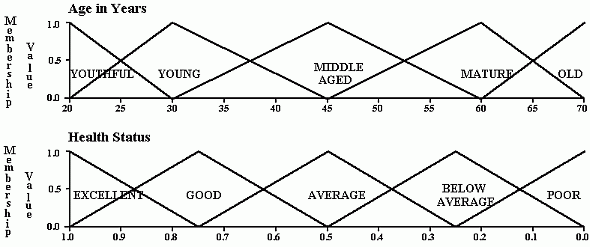Fuzzy logic: separable functions - products of one-dimensional factors: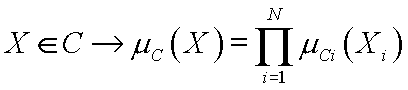Many other possibilities exist to produce N-dimensional membership functions.
One form of fuzzy rules is: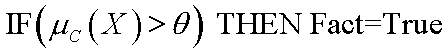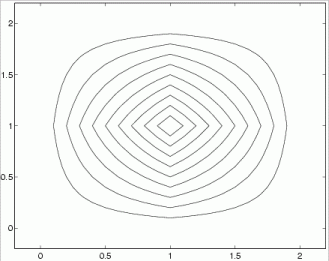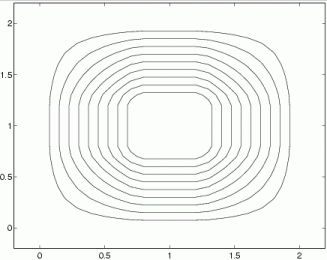Triangular and trapezoidal membership functions give such countours in 2D for different Th

Rough set logic

• find cases that certainly belong to the set
• find cases that certainly do not belong to the set
• all others are border cases with partial membership

Roughly: trapezoidal shapes of membership functions, but borders may be non-linear.

3. M-of-N rules

At least M conditions out of N are true.
Natural for neural systems, for example, if at least 2 logical conditions out of 4 are true: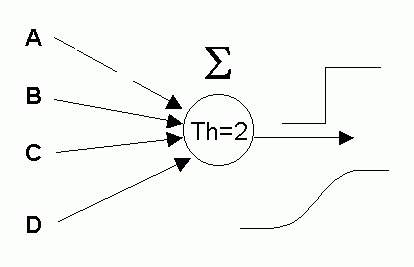IF at least 2 conditions out of {A,B,C,D} are true
THEN (X is a Brownie)
ELSE IF ... ELSE ...

 Clusters may have complex decision border shapes. IF (XÎC) THEN Factk = TRUE Granulation: covering with simpler shapes, corresponding to many rules. New buzz-word? Used for clusterization in the (input,output) space. Simple rules - non-linear feature transformations may be required. Neural networks, kernel methods (like SVM): nonlinear mapping from the feature space to the image space aiming at linear separability.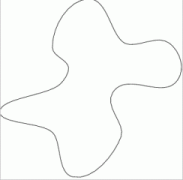Crisp logic rules are most desirable; try them first,
but remember ...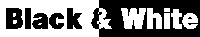• only one class is predicted P(Ci|X,M) = 0 or 1
black-and-white picture may be inappropriate in many applications• reliable crisp rules may reject some cases as unclassified
tradeoff: reliability (confidence in rules) - rejection rate

• discontinuous cost function allow only non-gradient optimization.

Fuzzy rules have continuous membership functions, giving some advantages:

• continuous classification probabilities P(Ci|X,M);
• all vectors classified (some with small probability);
• sometimes more natural than crisp rules.

But remember ...

• they are not so comprehensible as the crisp rules;

•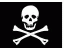danger of overparameterization - more complex rules, additional position/shape parameters.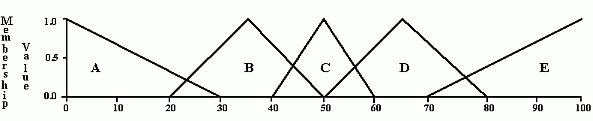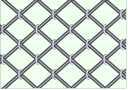• Fixed set of membership functions has less parameters, but
• fixing the MF shapes is a bad idea.
Curse of dimensionality:
k linguistic variables in d dimensions give kd areas.
Context-dependent linguistic variables - adapt membership functions in each rule.
Effect: clusters of different sizes at different input areas.

Problems with rule-based classification models:

• Interpretation of crisp rules may be misleading.
• Single crisp rule: prediction is unstable against small perturbations of input values.
• Rule-based classifiers: unstable sets of rules, small change in the dataset leads to a large change in structure of complex sets of rules.
• Fuzzy rules do not estimate real probabilities.
• How to find the best fuzziness/precision tradeoff ?

Knowledge accessible to humans:

• symbols and rules, crisp and fuzzy;
• similarity to prototypes;
• visualization - exploratory data analysis.

P-rules have the form: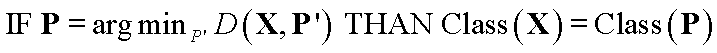D(X, P) is a distance function determining decision borders.

P-rules are easy to interpret!

IF X=You are most similar to the P=Superman THAN You are in the Super-league.
IF X=You are most similar to the P=Weakling THAN You are in the Failed-league.

In both cases “similar” may involve different features, i.e. different D(X, P) functions.

P-rules are more general than F-rules!
F-rules (and crisp rules) are P-rules with separable distance function. If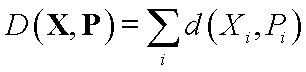for example, Manhattan function, then: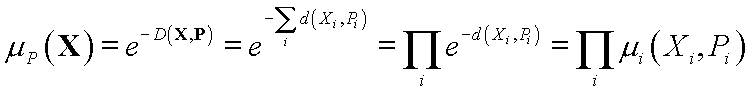If d(Xi, Pi) = 1 for Xi Î [Pi - D Pi , Pi + D Pi], and 0 outside, crisp rules are obtained.

Example: P-rules for Iris.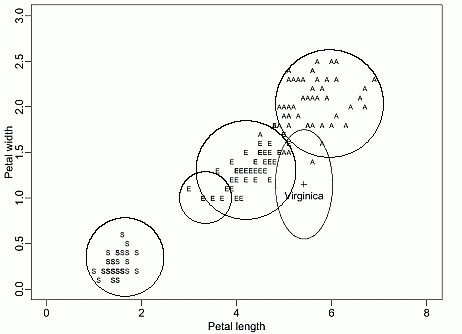First rule extraction/application is considered;
than some remarks on prototype-based and visualization-based methods are made.## Rule-based knowledge extraction methodology

Methodology of rule extraction, not a particular method.
Many decisions depend on particular application, not completely automatic.

Start from crisp rules - maybe they are sufficient?

1. Select linguistic variables sk(Xk,X'k) true if X in [Xk,X'k]; for discrete features define subsets.
• If the number of input feature is very high try feature selection methods first.
• Neural networks may aggregate several inputs providing new features.
• Histograms frequently provide a good start (smoothing is useful).
• For continuous features decision trees and neural networks perform automatic discretization.

• Simplest sets of rules with acceptable error should be found first; they are the most comprehensible.
• Sets of rules with growing complexity and accuracy may be found.
• Rules covering a few cases only are usually rejected but in some applications domain experts may find them useful.

3. Extract rules from data using neural, machine learning or statistical techniques.

4. Explore the reliability/rejection rate tradeoff while optimizing the rule set.

• Reliable rules make few errors but may reject some cases.
• Optimize linguistic variables (Xk,X'k intervals) using the rules extracted.

5. Repeat the procedure until a stable set of rules is found.
If linguistic variable have changed after optimization rule extraction may lead to different set of rules.

6. Find optimal degree of fuzzification to calculate probabilities and improve accuracy.
Fuzzification may be introduced during optimization.

## How to optimize sets of logical rules

Regularization of classification models (for example, network or tree pruning) allows to explore simplicity-accuracy tradeoff.
A set of rules M is obtained.

Next step: optimization of rules exploring the confidence-rejection rate tradeoff.

Define confusion matrix F(Ci,Cj|M) counting the number of cases from class Cj assigned by the set of rules M to the class Ci.

For 2 class problems:

F(Ci,Cj|M) =
 F++ F+- F-+ F--

where Fij is the frequency of cases Nij/N.
F(Ci,Cj|R) may be defined for a rule or a class.

Sensitivity of rules: Se=F++ / (F+++F+-) Î[0,1].
If Se=1 than all - cases (for example, sick) are never assigned to + class (for example, healthy).

Specificity of rules: Sp=F-- / (F-- + F-+) Î[0,1].
If Sp=1 than the rule never assigns healthy to sick.
These combinations are sometimes maximized (especially in medical statistics).

Rule confidence factor (relative frequency): Rc=F(Ci,Ci|R) / Sj F(Ci,Cj|R).
Rule support: how many cases does a rule cover?
Various ways of rule evaluation: entropy (information), m-relative frequency and other

Ideal: only the diagonal confusion matrix elements summing to N should be left.

• Minimize sum of the off-diagonals
• Maximize sum of the diagonals
• Combine the two.

Define weighted combination of the number of errors and the "predictive power" of rules: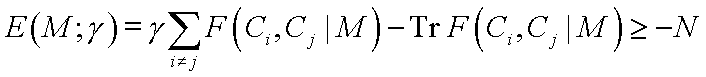This should be minimized without constraints; it is bound by -N (number of all training vectors).

Sets of rules M are parameterized by Xk, X'k intervals.
For g=0 predictive power of rules is maximized.
Rules that make fewer errors on the training data should be more reliable.
Cost function E(M; g) allows to reduce the number of errors to zero (large g) for rules M that reject some instances.

Optional risk matrix may be used: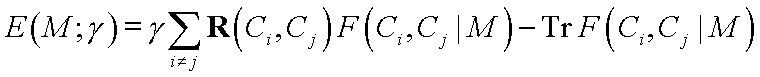Optimization of rules for a single class or linguistic variables for a single rule is possible.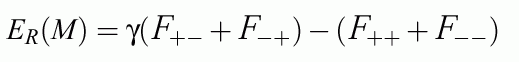Note: if the confusion matrix F(Ci,Cj|M) is discontinuous non-gradient minimization methods should be used (simplex, simulated annealing etc).

Result: sets of rules Mk of different reliability.
Frequently more accurate rule R(1) is contained in the less accurate rule R(2)

• use rule R(1) if it covers the case
• otherwise use rule R(2)

Reliability should be calculated for the border R(2) - R(1) only.
Estimations of reliability may be poor.

Example: Iris hierarchical rules

## How to use logical rules to calculate probabilities

Data from measurements/observations are not precise.
Finite resolution: Gaussian error distribution:

x => Gx=G(y;x,sx), where Gx is a Gaussian (fuzzy) number.

Given a set of logical rules {Â} apply them to input data {Gx }.
Use Monte Carlo sampling to recover p(Ci | X; {Â }) - this may be used with any classifier.

Analytical estimation of this probability is based on cumulant function: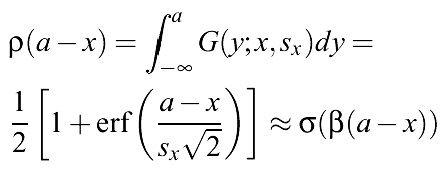Approximation better than 2% for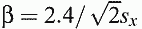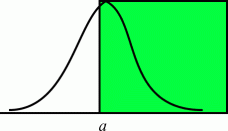The rule Ra(x) = {x>a} is true for Gx with probability: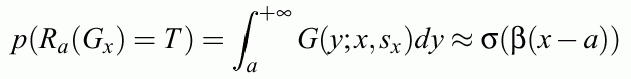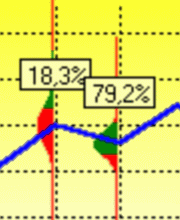If the logistic function is used instead of the error function the exact error distribution is
s(x)(1-s(x)); for s2=1.7 it is within 3.5% identical with Gauss.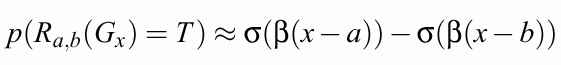Soft trapezoidal membership functions realized by L-units are obtained.
Fuzzy logic with such membership functions and crisp inputs is equivalent to crisp logic with Gx;
this is realized by MLP neural networks with logistic transfer functions.
MLP < = > FL with trapezoidal membership functions.

For conjunctive rule with many independent conditions:
R = r1 Ů r2 Ů ... rN the probability p(Ci |X) is a product of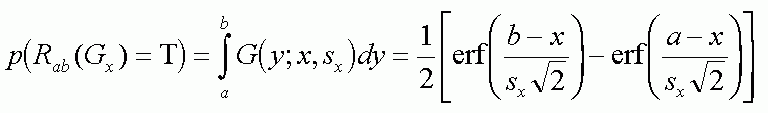If several rules cover the same input region: adding probabilities counts the overlapping regions many times. For two rules created for class C: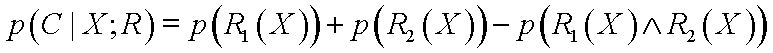This formula simply avoids counting the same regions twice. For more than 2 rules overlapping more terms appear!

This is not a fuzzy approach!
Here small receptive fields are used, in fuzzy approach typically 2-5 large receptive fields define linguistic variables.

Benefits:

1. Probabilities instead 0, 1 crisp rule decisions.
2. Vectors that were not classified by crisp rules have now non-zero probabilities.
3. Dispersions sx may be treated as adaptive parameters of the model M.
4. Gradient methods may be used for large-scale optimization.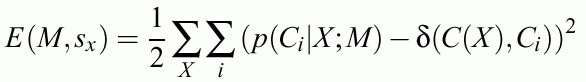Alternative approaches: flexible matching in machine learning.

## Neural rule extraction methods developed in our group

Several practical rule-extraction methods developed in our group:

### 1. Modified constrained constructive C-MLP2LN method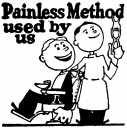Simplify the network leaving only 0, ±1 weights, use special linguistic units for input discretization.

### 2. Search-based MLP method (S-MLP)

Use integer weights/biases.
Start from Wij = 0, biasi = -0.5, change by 1.
Use beam search techniques instead of backpropagation.

### 3. FSM, Feature Space Mapping density estimating network

FSM (Feature Space Mapping) - separable transfer functions, neurofuzzy network.
Crisp rules: FSM + rectangular transfer functions.
Fuzzy rules: FSM + context-dependent fuzzy membership functions.
Localized functions may be treated as prototypes.

## Overview of decision-tree based methods

### 4. SSV, Separability Split Value decision tree

SSV separability criterion: separate maximum number of pairs from different classes minimizing the number of separated pairs from the same class.

## Prototype-based explanation

Select the best prototypes - "supermans".

## Visualization-based explanationExplanatory data analysis - show the data.
Overview of visualization methods: if time permits ...
SOM - most popular, trying to classify/display at the same time, but poorly.

### 6. PCI, Probabilistic Confidence Intervals

May be used with any classifier.
Shows the probabilities in the neighborhood of the case analyzed for all/each feature.
Allows to evaluate reliability of classification, but not to explain the data.

### 7. IMDS, Interactive MultiDimensional Scaling

Used directly on the data.
Shows interactively the neighborhood of the case analyzed preserving topographic relations.

### 8. GhostMiner: all this and more

Just a brief mention of GhostMiner, our data mining system developed in collaboration with Fujitsu Kyushu Ltd (FQS).

## Some knowledge discovered

Results for many datasets illustrating the methodology described above.

### Analysis of psychometric questionnaires

Showing an example of a system based on rules derived from the data.

## Open problems

In real world projects training and finding optimal networks is not our hardest problem ...
Good methods to discover rules exist although proving that simplest sets of rules have been discovered is usually not possible.

Discovering hierarchical structure in the data:

• basic tests are performed first and hypothesis made;
• only the tests necessary to confirm initial hypothesis are made;
• if confirmed no further tests are made; if not more tests are made;
• the data contain large groups of missing values.

Dealing with unknown values.

• values that are not known or have been corrupted in the measurement process (questions not answered);
• values that have not been measured on purpose (questions not asked).

Constructing new, more useful features, from hundreds of 'weak' features.
Constructing the simplest explanations for the data.
Constructing theories that allow to reason about data.
Constructing new and modifying existing classes.
Building complex systems interacting with humans.

### References

IEEE Transactions on Neural Networks 12 (2001) 277-306

Most papers are available from these pages
https://www.fizyka.umk.pl/kmk/publications.html
https://www.is.umk.pl/~duch/cv/papall.html